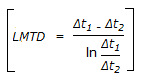# E-PolyLearning

## Discussion Forum

 Que. Out of the following four assumptions used in the derivation of the equation for LMTD given by below equation, which one is subject to the largest deviation in practice ?a. Constant overall heat transfer co-efficient. b. Constant rate of fluid flow. c. Constant specific heat. d. No partial phase change in the system.
 Correct Answer:Constant rate of fluid flow.